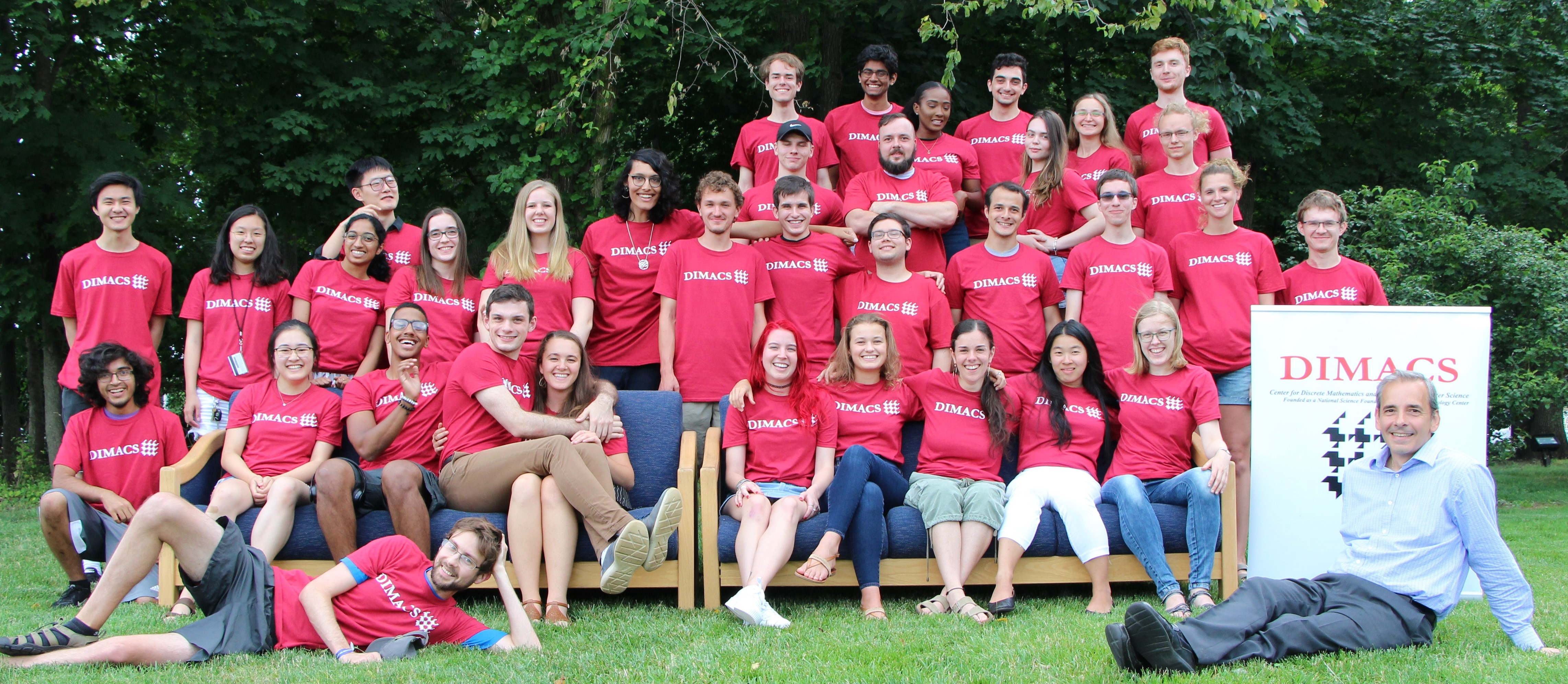Research Experiences for Undergraduates 2019

May 28 - July 21, 2019, Rutgers, Piscataway, NJ, USA

July 21 - August 2, 2019, Charles University, Prague, Czech RepublicResearch projects of the Czech group

Random walks with excited vertices

Mentor: Bhargav Narayanan

Students: Petr Chmel, Jan Petr, Mikhail Beliyeu

When considering simple random walks on a graph, it is known that the expected hitting time has the upper bound of approximately O(n^3). However, the random walker may use some heuristics or biases to try to get to the target vertex quicker.

One of such biases is 'geodesic bias' - some vertices in the graph are 'excited' and from them, the random walker takes a step along a fixed shortest path. The question is, do the upper bounds differ?

Space-Time-Depth

Mentor: Periklis Papakonstantinou

Students: Pavel Dvořák, Kyle Hess, Lukáš Ondráček, Jakub Pekárek

Let M be a Turing machine working in time T(n). Trivial upper bound for used space by M is T(n). There is constructions of Turing machine M' such that M' is equivalent to M and it uses only T(n)/log T(n) space. There is also a construction of circuit C equivalent to M such that the depth of the circuit is T(n). Can we do better?

Rainbow cycles

Mentor: Sophie Spirkl

Students: Petra Pelikánová, Aneta Šťastná

The main goal of this project is to obtain some partial result of the following conjecture by Aharoni: Let G be an n-vertex graph and let {E_1,...,E_n} be a partition of its edge set with |E_i| <= k for all i in [n] and for some natural number k. Then, G has a rainbow cycle of length at most ceiling of n/k.

This conjecture generalizes conjecture of Caccetta-Häggkvist: Let G be an n-vertex directed graph such that every vertex has out-degree at least k. Then, G has a directed cycle of length at most ceiling of n/k.

Ramsey-like game

Mentor: Sophie Spirkl

Students: Andrej Dedík, Jan Soukup

Consider the following game. It is played by two players on an intially empty discrete graph. One player tries to force induced subgraph (which is given at the start of the game) by choosing stable sets, into which the other player has to draw edges. The forcing (player one) player wins if he forces second player to complete the induced subgraph. Other player wins if forcing player fails to do so. The question which pops up is: Is it always possible to force graph on some given number of vertices? This question has been answered, and as it turns out, the answer is yes - for each graph there is such a number of vertices, such that forcing player has strategy to force the graph in question. What we do not know though, is what this number is for most of the known graphs. We know, that for complete graphs the number is (for obvious reasons) Ramsey's number, but not much else is known. There is an lower and upper bound, but they are really quite far from each other. Hence our goal is to get a deeper understanding of this problem and investigate if these bounds could be squeezed together more tightly.

Prague part of REU

Conference during the Charles University program:

FundingThis project has received funding from the European Union's Horizon 2020 research and innovation programme under the Marie Skłodowska-Curie grant agreement No 823748.

Senior organizer: Martin Loebl
Graduate coordinators: Pavel Koblich Dvořák, Jakub Pekárek

Past REUs

Webmaster: kamweb@kam.mff.cuni.cz         Modified: 03. 10. 2019